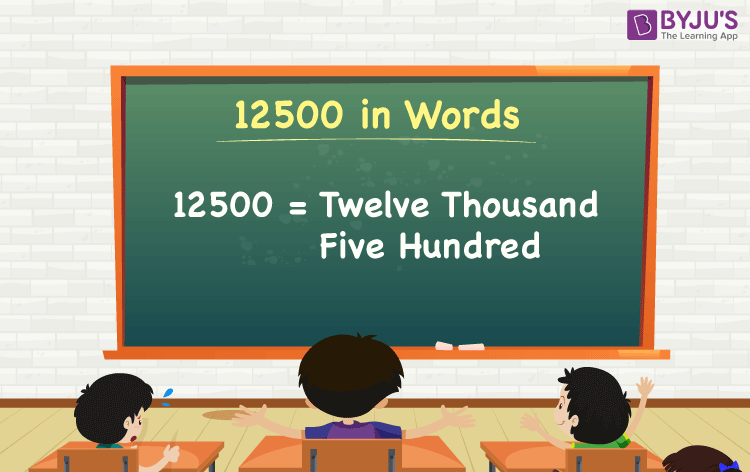12500 = Twelve thousand five hundred

We can write 12500 in words as Twelve thousand five hundred. If you paid Rs. 12500 to buy a new smartwatch, you can say to your friend, “I bought a smartwatch worth Twelve thousand five hundred rupees”. Also, we know that 12500 is a cardinal number since it represents a certain amount.

 12500 in words: Twelve thousand five hundred Twelve thousand five hundred in numerical form: 12500

12500 in English Words

Generally, we use the English alphabet to write numbers in words and thus, we can write 12500 in English words as “Twelve thousand five hundred”.## How to Write 12500 in Words?

We can convert 12500 to words using a place value chart. This can be done as follows.

12500 is a five-digit number, so let’s make a table that contains the place value up to 5 digits.

 Ten thousand Thousands Hundreds Tens Ones 1 2 5 0 0

Let us expand the digits using their place values written in the above table.

= 1 × Ten thousand + 2 × Thousand + 5 × Hundred + 0 × Ten + 0 × One

= 1 × 10000 + 2 × 1000 + 5 × 100 + 0 × 10 + 0 × 1

= 10000 + 2000 + 500

= 12000 + 500

= 12500

= Twelve thousand five hundred

Interesting way of writing 12500 in words:

1 = One

12 = Twelve

120 = One Hundred and twenty

125 = One hundred and twenty-five

1250 = One thousand two hundred and fifty

12500 = Twelve thousand five hundred

12500 is a natural number that is the successor of 12499 and predecessor of 12501.

12500 in words – Twelve thousand five hundred

Is 12500 an odd number? – No

Is 12500 an even number? – Yes

Is 12500 a prime number? – No

Is 12500 a composite number? – Yes

Is 12500 a perfect square number? – No

Is 12500 a perfect cube number? – No

## Frequently Asked Questions on 12500 in Words

Q1

### How do you write 12500 in words?

We can write 12500 in words as Twelve thousand five hundred.

Q2

### How do you write 12500 in words on a cheque?

On a cheque, we generally write 12500 in words as Twelve thousand five hundred rupees only.

Q3

### Write the value of 10000 + 2500 in words.

10000 + 2500 = 12500
Therefore, the sum of ten thousand and two thousand five hundred is Twelve thousand five hundred.

Test your Knowledge on 12500 in Words